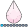## GTL conditional logic question

I have a GTL template question on conditional formatting.  I have a series of graphs I render with SGRENDER with a BY statement.  Let’s say my BY statement looks like

BY var1 var2 var3;

VAR2 has a range from 0 to 5.  When it is 0 (zero) I want to draw a different graph.  I tried using DYNAMIC variables but that didn’t do it.

Is there a way I can do this?

Thanks,

Jim Horne

1 ACCEPTED SOLUTION

Accepted Solutionsdjrisks
Barite | Level 11

## Re: GTL conditional logic question

Hi Jim,

I know your question has already been answered by Rick and for future reference, if you did want to create a flexible template than you need to use the _byvar_ and _byval_ dynamic variables in your template. This will allow you to do different graphs dependent on your data.

Below is an example, and you can see that the template is using if (_byval2_ = 0) instead of if (cyclinders = 0). This is a very important part of the conditional statement.

``````
data cars;
set sashelp.cars;
where make = "Audi";
if Cylinders = 6 then Cylinders = 0;
run;

proc sort data = cars;
by make Cylinders;
run;

proc template;
define statgraph cond;
dynamic _byval_ _byvar_ _byval2_ _byvar2_;
begingraph;
if (_byval2_ = 0) /* Plot for 0 condition */
layout overlay;
barchart x = make y = horsepower / stat = sum fillattrs = (color=red);
endlayout;
else
layout overlay;
barchart x = make y = _byvar2_ / fillattrs = (color=blue);
endlayout;
endif;
endgraph;
end;
run;

proc sgrender data = cars template=cond;
by make cylinders;
run;

``````

Thanks.

8 REPLIES 8

## Re: GTL conditional logic question

I suggest you write two templates and call them conditionally from PROC SGRENDER by using a WHERE clause:

``````proc sgrender data=Have template=TmplNonZero;
where var2 > 0;
by var1 var2 var3;
run;

proc sgrender data=Have template=TmplZero;
where var2 = 0;
by var1 var2 var3;
run;``````

## Re: GTL conditional logic question

Rick,

Thanks, I had not thought of that.  I can make it work although I'll have to play games to get my drill down pointers to work.  I'll also have to make two passes through the data which I would rather avoid.  My SGRENDER currently takes about 3 minutes because of the sheer number of observations it reads.

I'm not complaining because you have given me a solution - which I didn't have.  I just was hoping for something more elegant.  But this runs in batch at night so it should not be a big deal.

Thanks again,

Jim

## Re: GTL conditional logic question

I would be surprised if this lengthens the execution time by much. Reading the data is usually much faster than creating the graphs.  It might even speed things up, since each call to SGRENDER has less data to process. Report back on how long it takes after you get it working.Jay54
Meteorite | Level 14

## Re: GTL conditional logic question

Three minutes?  I would like to see this use case with data to see what is taking so long.

## Re: GTL conditional logic question

RIck and Sanjay,

Thanks for the replies.  Rick, you were right, run time did not go up at all.  It may have actually taken a few seconds off the run time.  In testing, that seems to be the case.  Sanjay, I don't know if you need to see the data or just to know that a total of over 200 graphs are produced.  This mornings runs (production and my test split out) each took about two minutes 20 seconds in the SGRENDER steps for this work.  Since each graph is a four part panel this may be why it takes the time it does.

Jim Hornedjrisks
Barite | Level 11

## Re: GTL conditional logic question

Hi Jim,

I know your question has already been answered by Rick and for future reference, if you did want to create a flexible template than you need to use the _byvar_ and _byval_ dynamic variables in your template. This will allow you to do different graphs dependent on your data.

Below is an example, and you can see that the template is using if (_byval2_ = 0) instead of if (cyclinders = 0). This is a very important part of the conditional statement.

``````
data cars;
set sashelp.cars;
where make = "Audi";
if Cylinders = 6 then Cylinders = 0;
run;

proc sort data = cars;
by make Cylinders;
run;

proc template;
define statgraph cond;
dynamic _byval_ _byvar_ _byval2_ _byvar2_;
begingraph;
if (_byval2_ = 0) /* Plot for 0 condition */
layout overlay;
barchart x = make y = horsepower / stat = sum fillattrs = (color=red);
endlayout;
else
layout overlay;
barchart x = make y = _byvar2_ / fillattrs = (color=blue);
endlayout;
endif;
endgraph;
end;
run;

proc sgrender data = cars template=cond;
by make cylinders;
run;

``````

Thanks.

## Re: GTL conditional logic question

That's perfect.  It only required adding three lines to my existing production teplate - the DYNMIC statement for the _BYVAL_ and _BYVALn_ variables, and the IF - ENDIF construct around the cell that cannot write data if the BY varoable I check = 0.

That's elegant - and easy to maintain.  I wish the automtic variables were easier to find.  When I read your post I Googled "SAS DYNAMIC _BYVAL_" and found it in the GTL User Guide buried in a section I managed to overlook.djrisks
Barite | Level 11